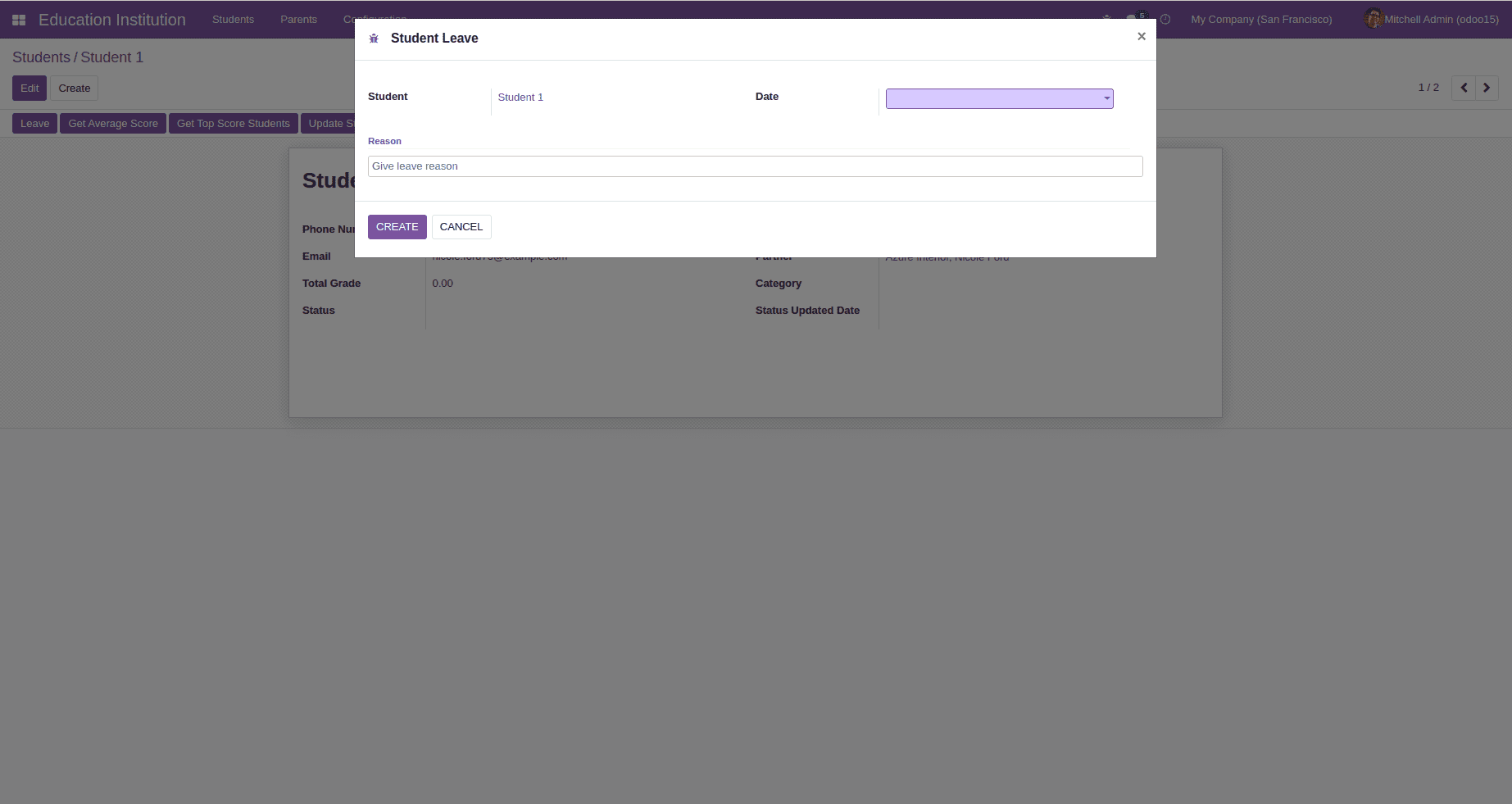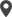# Chapter 4 - Odoo 15 Development Book

## Wizard

Wizards in odoo are used for creating interactive sections with users. For any business flow, such interactive sections are very useful.Transient and Abstract models are mainly used model class in Odoo for creating wizard. however Transient mode is the commonly used one.This gives all the features of a model class exept that the data in a Transient model is deleted periodically, hence the name Transient.Wizards are commonly used to perform operations on database persistent models.

Let us discuss the use case with an example. Consider a model student.student for managing all the student records of an educational organization.

``````from odoo import fields, models, api
class Student(models.Model):
_name = "student.student"
_description = "Student"
name = fields.Char(string="Name", required=True)
partner_id = fields.Many2one('res.partner', string="Partner")
phone = fields.Char(string="Phone Number")
email = fields.Char(string="Email", required=True)
status = fields.Char(string="Status")
leave_ids = fields.One2many('student.leave', 'student_id', string="Leaves")``````

There is a one2many type field for recording all leaves taken by the student. Let’s create the model student.leave with required fields.

``````class StudentLeave(models.Model):
_name = "student.leave"
_description = "Student Leave"
student_id = fields.Many2one('student.student', string="Student")
date = fields.Date(string="Date")
reason = fields.Char(string="Reason")``````

Then let’s create the wizard model from which the user can pick a date and add reason for leave. Transient model class is used here for the wizard model.

``````from odoo import api, fields, models
class StudentLeaveWizard(models.TransientModel):
_name = 'student.leave.wizard'
_description = 'Student Leave'
student_id = fields.Many2one('student.student', string="Student",
date = fields.Date(string="Date")
reason = fields.Char(string="Reason")``````

Now add a button inside the student form view for creating a leave record.

``````<header >
<button name="create_leave" string="Leave" class="oe_highlight"
type="object"/>

Write python function for the button action in the student model. Lets return a widget in which user can select the date and give the reason for leave. Use this widget values for creating a new leave record for the student. For that, create a new record in the widget model with student_id field value set as the current student.Then return that object in the button click.

``````def create_leave(self):
wizard = self.env['student.leave.wizard'].create({
'student_id': self.id
})
return {
'name': _('Student Leave'),
'type': 'ir.actions.act_window',
'res_model': 'student.leave.wizard',
'view_mode': 'form',
'res_id': wizard.id,
'target': 'new'
}``````

Next step is to define form view for the wizard with buttons to perform actions on the record. Here 2 buttons named CREATE and CANCEL are added for the wizard view each having functionalities same as the name indicates.

``````<record model="ir.ui.view" id="leave_wizard_form_view">
<field name="name">Leave</field>
<field name="model">student.leave.wizard</field>
<field name="arch" type="xml">
<form string="Leave">
<sheet>
<group>
<group>
<field name="student_id"/>
</group>
<group>
<field name="date" required="1"/>
</group>
</group>
<separator string="Reason"/>
<field name="reason" required="1" nolabel="1" placeholder="Give leave reason"/>
</sheet>
<footer>
<button type="object" name="create_leave_from_wizard" class="btn btn-primary"
string="CREATE"/>
<button string="CANCEL" special="cancel"/>
</footer>
</form>
</field>
</record> ``````

This will return the widget form on button click.Now perform the required action inside the create_leave_from_wizardm ethod.This method has to be written inside the wizard model. Create a new record in the model student.leave using widget value on the button click.

``````def create_leave_from_wizard(self):
self.env['student.leave'].create({
'student_id': self.student_id.id,
'date': self.date,
'reason': self.reason
})``````

After performing all the changes, upgrade the module and check how a new leave record is created for the student.This is how a wizard is created which will improve the overall user experience.Calicut

Cybrosys Technologies Pvt. Ltd.
Neospace, Kinfra Techno Park
Kakkancherry, Calicut
Kerala, India - 673635London

Cybrosys Limited
Alpha House,
100 Borough High Street, London,
SE1 1LB, United KingdomKochi

Cybrosys Technologies Pvt. Ltd.
1st Floor, Thapasya Building,
Kochi, India - 682030.Bangalore

Cybrosys Techno Solutions
The Estate, 8th Floor,